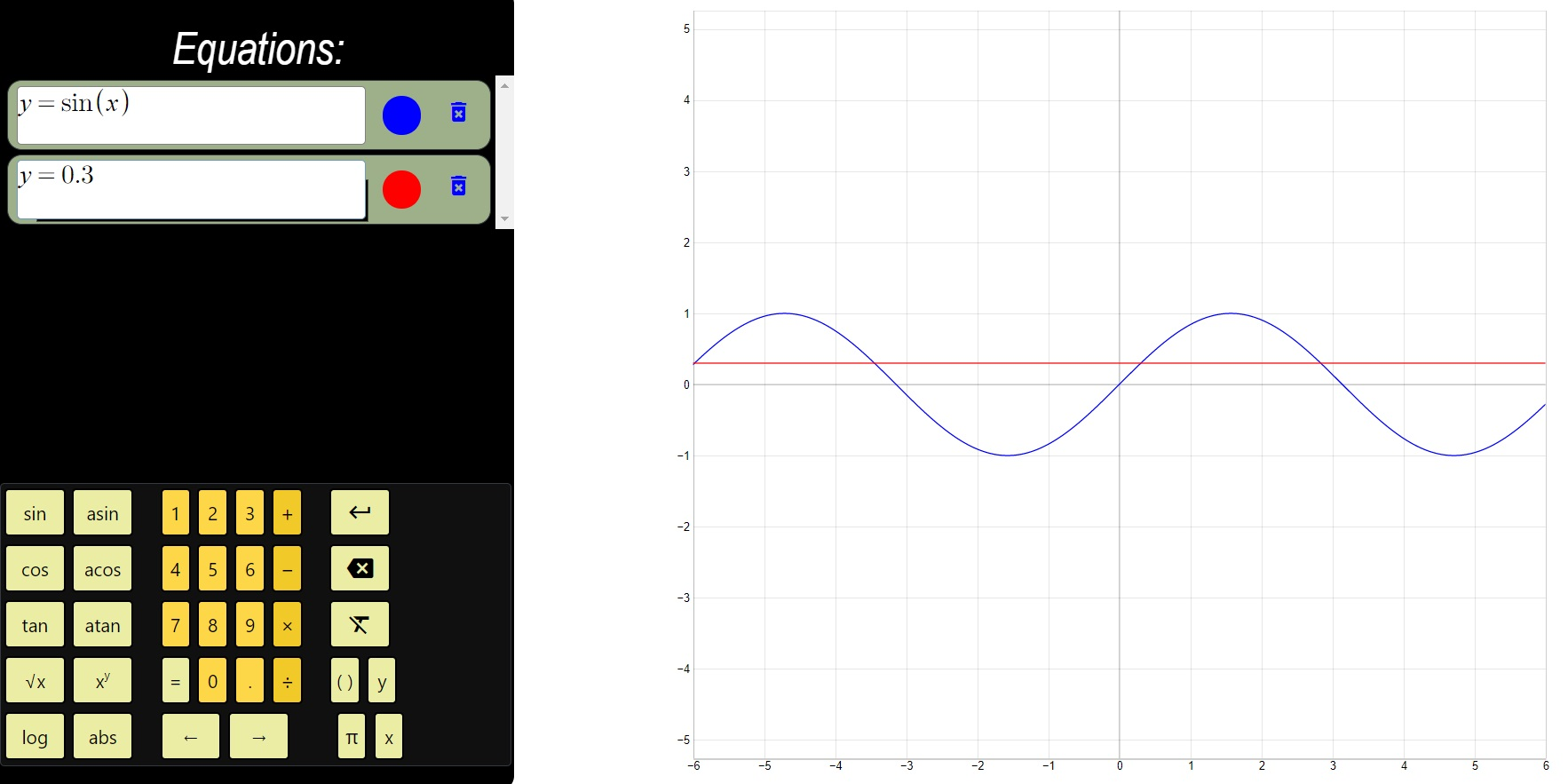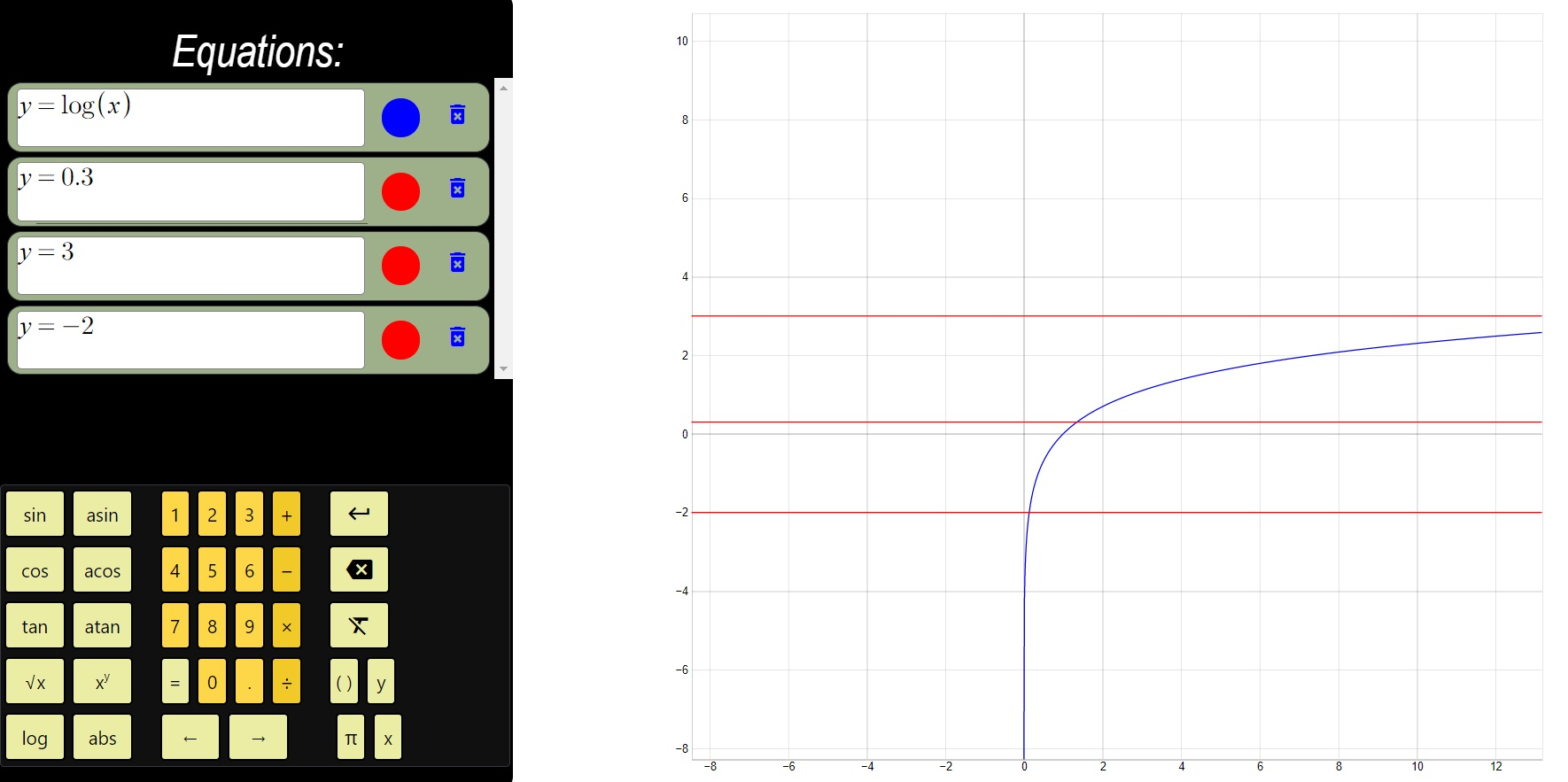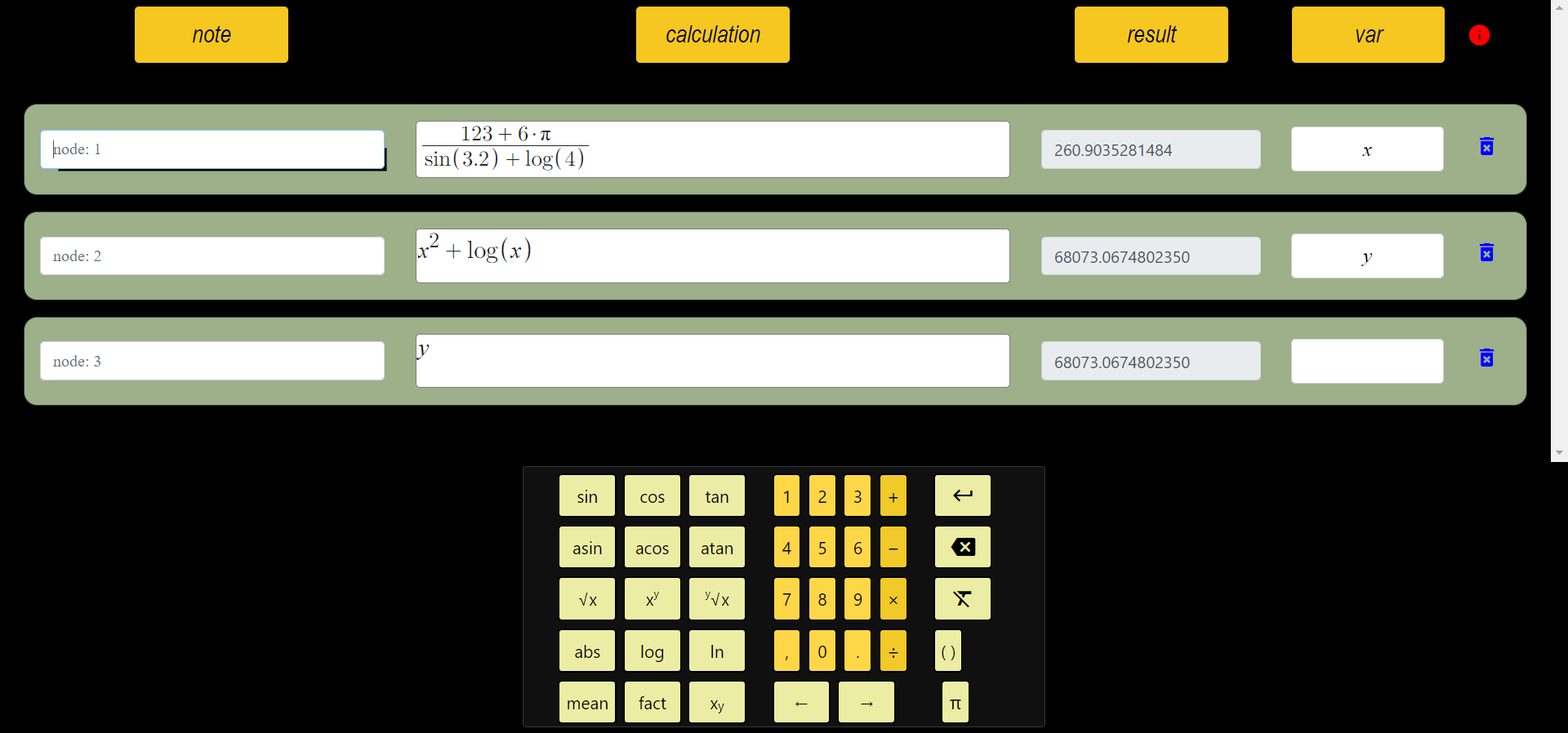# One-to-One Function

For non-empty sets A and B f : If the function value of each different element in the domain of the function A → B is different, the function f is called a one-to-one function. For ∀a, b ∈ A, f is one-to-one if the condition a ≠ b ⇒ f(a) ≠ f(b) or f(a) = f(b) ⇒ a = b is met.
In other words, if f(a)=f(b) equality can be achieved for any two different values ​​in the domain (let it be a, b), the function is not one-to-one. Another way to understand this is to look at the graph of the function:Since a parallel (red) drawn to the x-axis in the above graph can intersect the f(x) = sin(x) function (blue) at more than one point, the function is not one-to-one. However, since it is not possible to draw a line parallel to the x-axis and intersecting f(x) = log(x) at more than one point in the graph below, the function is one-to-one.Click to draw function graphs.
Non-one-to-one functions are functions that cannot be reversed because: Let f(x) be a non-one-to-one function. So it is possible to find values ​​of a and b that are not equal to each other and satisfy f(a) = f(b). Let g(x) be the inverse of f(x). g(f(a)) = a and g(f(b)) = b. However, the equation g(f(a)) = g(f(b)) = a = b cannot be true because the values ​​of a and b are different from each other. Thus, we prove by contradiction that non-one-to-one functions are not reversible for the entire domain. In the range in which they are reversible, there should not be different values ​​of a and b, which satisfy the equation f(a) = f(b).

###### All Articles

id: PucnQqJgST